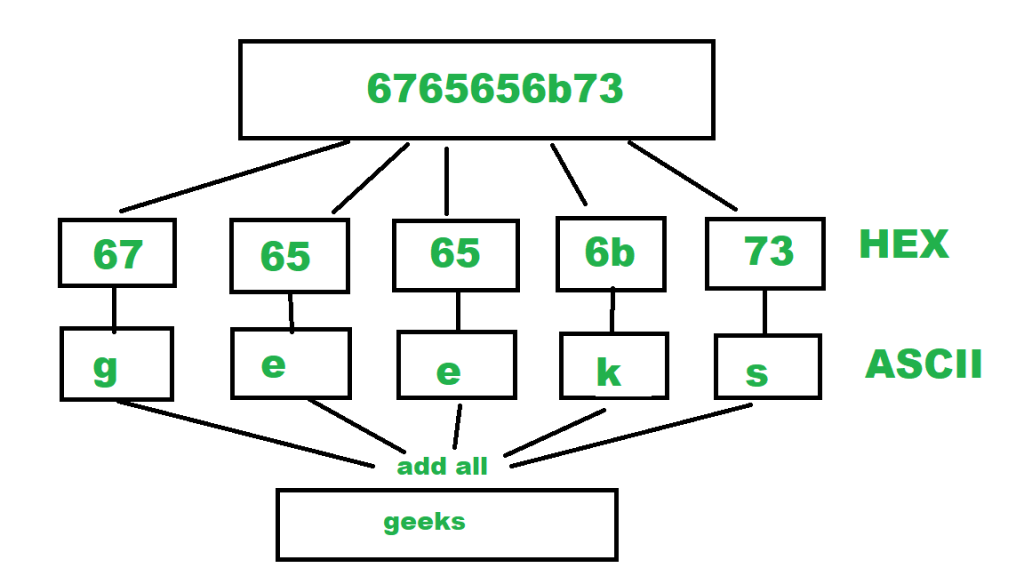# Convert Hexadecimal value String to ASCII value String

Given Hexadecimal value string as input, the task is to convert the given hexadecimal value string into their corresponding ASCII format string.

Examples:

```Input: 6765656b73
Output: geeks

Input:  6176656e67657273
Output: avengers
```

## Recommended: Please try your approach on {IDE} first, before moving on to the solution.

The “Hexadecimal” or simply “Hex” numbering system uses the Base of 16 system. Being a Base-16 system, there are 16 possible digit symbols. Hexadecimal number uses 16 symbols {0, 1, 2, 4, 5, 6, 7, 8, 9, A, B, C, D, E, F} to represent all numbers. Here, (A, B, C, D, E, F) represents (10, 11, 12, 13, 14, 15).
ASCII stands for American Standard Code for Information Interchange. ASCII is a standard that assigns letters, numbers, and other characters within the 256 slots available in the 8-bit code. E.g the lower case “h” character (Char) has a decimal value of 104, which is “01101000” in binary and “68” in hexadecimal.
For more ASCII values, refer ASCII TABLE.

Algorithm:

1. Initialize final ascii string as empty.
2. Extract first two characters from the hexadecimal string taken as input.
3. Convert it into base 16 integer.
4. Cast this integer to character which is ASCII equivalent of 2 char hex.
5. Add this character to final string.
6. Extract next two characters from hexadecimal string and go to step 3.## C++

 `// C++ program to convert hexadecimal ` `// string to ASCII format string ` `#include ` `using` `namespace` `std; ` ` `  `string hexToASCII(string hex) ` `{ ` `    ``// initialize the ASCII code string as empty. ` `    ``string ascii = ``""``; ` `    ``for` `(``size_t` `i = 0; i < hex.length(); i += 2) ` `    ``{ ` `        ``// extract two characters from hex string ` `        ``string part = hex.substr(i, 2); ` ` `  `        ``// change it into base 16 and  ` `        ``// typecast as the character ` `        ``char` `ch = stoul(part, nullptr, 16); ` ` `  `        ``// add this char to final ASCII string ` `        ``ascii += ch; ` `    ``} ` `    ``return` `ascii; ` `} ` ` `  `// Driver Code ` `int` `main() ` `{ ` `    ``// print the ASCII string. ` `    ``cout << hexToASCII(``"6765656b73"``) << endl; ` ` `  `    ``return` `0; ` `} ` ` `  `// This code is contributed by ` `// sanjeev2552 `

## Java

 `// Java program to convert hexadecimal ` `// string to ASCII format string ` `import` `java.util.Scanner; ` ` `  `public` `class` `HexadecimalToASCII { ` ` `  `    ``public` `static` `String hexToASCII(String hex) ` `    ``{ ` `        ``// initialize the ASCII code string as empty. ` `        ``String ascii = ``""``; ` ` `  `        ``for` `(``int` `i = ``0``; i < hex.length(); i += ``2``) { ` ` `  `            ``// extract two characters from hex string ` `            ``String part = hex.substring(i, i + ``2``); ` ` `  `            ``// change it into base 16 and typecast as the character ` `            ``char` `ch = (``char``)Integer.parseInt(part, ``16``); ` ` `  `            ``// add this char to final ASCII string ` `            ``ascii = ascii + ch; ` `        ``} ` ` `  `        ``return` `ascii; ` `    ``} ` `    ``public` `static` `void` `main(String[] args) ` `    ``{ ` `        ``// print the ASCII string. ` `        ``System.out.println(hexToASCII(``"6765656b73"``)); ` `    ``} ` `} `

## Python3

 `# Python3 program to convert hexadecimal  ` `# string to ASCII format string ` ` `  `def` `hexToASCII(hexx): ` ` `  `    ``# initialize the ASCII code string as empty. ` `    ``asci ``=` `"" ` ` `  `    ``for` `i ``in` `range``(``0``, ``len``(hexx), ``2``): ` ` `  `        ``# extract two characters from hex string ` `        ``part ``=` `hexx[i : i ``+` `2``] ` ` `  `        ``# change it into base 16 and ` `        ``# typecast as the character  ` `        ``ch ``=` `chr``(``int``(part, ``16``)) ` ` `  `        ``# add this char to final ASCII string ` `        ``asci ``+``=` `ch ` `     `  `    ``return` `asci ` ` `  `# Driver Code ` `if` `__name__ ``=``=` `"__main__"``: ` ` `  `    ``# print the ASCII string. ` `    ``print``(hexToASCII(``"6765656b73"``)) ` ` `  `# This code is contributed by ` `# sanjeev2552 `

## C#

 `// C# program to convert hexadecimal ` `// string to ASCII format string ` `using` `System; ` ` `  `class` `GFG  ` `{ ` `    ``public` `static` `String hexToASCII(String hex) ` `    ``{ ` `        ``// initialize the ASCII code string as empty. ` `        ``String ascii = ``""``; ` ` `  `        ``for` `(``int` `i = 0; i < hex.Length; i += 2) ` `        ``{ ` ` `  `            ``// extract two characters from hex string ` `            ``String part = hex.Substring(i, 2); ` ` `  `            ``// change it into base 16 and  ` `            ``// typecast as the character ` `            ``char` `ch = (``char``)Convert.ToInt32(part, 16);; ` ` `  `            ``// add this char to final ASCII string ` `            ``ascii = ascii + ch; ` `        ``} ` `        ``return` `ascii; ` `    ``} ` `     `  `    ``// Driver Code ` `    ``public` `static` `void` `Main(String[] args) ` `    ``{ ` `        ``// print the ASCII string. ` `        ``Console.WriteLine(hexToASCII(``"6765656b73"``)); ` `    ``} ` `} ` ` `  `// This code is contributed by PrinciRaj1992 `

Output:

```geeks
```

My Personal Notes arrow_drop_upI am a Developer I love to code and bring my ideas alive

If you like GeeksforGeeks and would like to contribute, you can also write an article using contribute.geeksforgeeks.org or mail your article to contribute@geeksforgeeks.org. See your article appearing on the GeeksforGeeks main page and help other Geeks.

Please Improve this article if you find anything incorrect by clicking on the "Improve Article" button below.

Improved By : sanjeev2552, princiraj1992

Article Tags :
Practice Tags :

Be the First to upvote.

Please write to us at contribute@geeksforgeeks.org to report any issue with the above content.